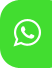## Product Detail## Ignou Solved Assignment For Che 01 Atoms and Molecules

University  IGNOU
Service Type Solved Assignment
Course BA(Chemistry)
Semester
Short Name or Subject Code Che 01 Atoms and Molecules
Product BA(Chemistry) of Solved Assignment (IGNOU)
Pattern
Price
Click to view price

Attempt all the questions. Each question carries 10 marks.

1. a) For the first two lines of Balmer series, identify the values of n1 and n2.

b) Calculate the ionisation energy of hydrogen atom using Bohr’s theory.

c) Calculate the energy values of the electron in second and third orbits of hydrogen atom.

2. a) The first ionisation energies of silicon and sulphur are lower than that of phosphorus. Explain.

b) Explain use of cation to anion radius ratio.

c) Arrive at the Lewis structures of   5 4 SnCl using the steps indicated in Sec. 3.7 of and IF Unit 3.

d) The bond enthalpies of N–N, N=N and N≡N bonds are not in the ratio 1:2:3. Explain the reason.

3. a) Using Pauli’s exclusion principle, work out the total number of electrons in the level n=3.

b) For a particle in one-dimensional box, write the equations for calculating the energy values corresponding to

i) n = 1

ii) n = 2

iii) n = 3

c) Calculate the de Broglie wavelength associated with a ball of mass 0.50 kg moving with a velocity of 20 m s−1 .

d) What is a well-behaved wave function?

4. a) State the definitions of bonding, anti-bonding and nonbonding orbitals. Draw the molecular orbitals obtained by the linear combination of two 1s orbitals.

b) Write down the molecular orbital configurations of N2 and NO. Calculate their bond orders. Which of these two is expected to have paramagnetic behavior?

5. a) Assuming that the covalent radius of hydrogen is 28 pm when it is bonded to other atoms, and using Table 3.10, calculate the bond lengths of the bonds in CH2 = CH−CH2−NH2

b) Alkyl halides are more reactive than alkanes. Explain the reason.

c)  3 I ion is known to exist but not  F ion. Explain the reason after assigning Lewis 3 structures for both the ions.

6. a) Write down the structures of stereoisomers of 2, 3–pentanediol. Identify the enantiomeric pairs.

b) State the difference between the racemic mixture and meso form.

c) Suggest a method of determination of molar extinction coefficient.

7. a) Write the resonance structures of formate ion. What do you infer regarding the relative bond length values of two C−O bonds in it? Explain the reason.

b) Predict the hybridisation state of each carbon atom in the following compounds:

CH3 CH2 C CH C C CH3 CH2 CH C O O CH3 CO2

8. a) The rate constant for Cr decay is 2.89×10−7 s−1. Calculate the time required for 87.5% decay.

b) Explain the method of determining the age of an organic material.

c) Explain the following terms: i) Moderator and ii) Breeder reactor.

9. a) Among the molecules given below, identify those which are microwave active. Br2, HF, CO2 and CO

b) Write down the values of two characteristic frequencies for each of the following compounds:

i) C CH

ii) H3C CH3 OH

iii) H3C H

c) State the condition to be satisfied for a molecule to absorb in the microwave region.

10. a) Give an example for each of the following types of nuclear transmutation reactions:

(p, α), (n, p), ( n, α) and (p, n).

b) An analyst is asked to oxidize a secondary alcohol to ketone. What single characteristic feature in IR spectra should the analyst look for in order to verify the feasibility of the reaction?

c) Which of the following has higher λmax value? 1-butene or 1, 3 – butadiene. State the reason.